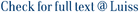Several well known integral stochastic orders (like the convex order, the supermodular order, etc.) can be defined in terms of the Hessian matrix of a class of functions. Here we consider a generic Hessian order, i.e., an integral stochastic order defined through a convex cone of Hessian matrices, and we prove that if two random vectors are ordered by the Hessian order, then their means are equal and the difference of their covariance matrices belongs to the dual of . Then we show that the same conditions are also sufficient for multinormal random vectors. We study several particular cases of this general result.

Hessian orders and multinormal distributions / Arlotto, A; Scarsini, Marco. - In: JOURNAL OF MULTIVARIATE ANALYSIS. - ISSN 0047-259X. - 100:(2009), pp. 2324-2330. [10.1016/j.jmva.2009.03.009]

### Hessian orders and multinormal distributions

#### Abstract

Several well known integral stochastic orders (like the convex order, the supermodular order, etc.) can be defined in terms of the Hessian matrix of a class of functions. Here we consider a generic Hessian order, i.e., an integral stochastic order defined through a convex cone of Hessian matrices, and we prove that if two random vectors are ordered by the Hessian order, then their means are equal and the difference of their covariance matrices belongs to the dual of . Then we show that the same conditions are also sufficient for multinormal random vectors. We study several particular cases of this general result.
##### Scheda breve Scheda completa Scheda completa (DC)2009
Hessian orders and multinormal distributions / Arlotto, A; Scarsini, Marco. - In: JOURNAL OF MULTIVARIATE ANALYSIS. - ISSN 0047-259X. - 100:(2009), pp. 2324-2330. [10.1016/j.jmva.2009.03.009]
File in questo prodotto:
File
JMVA2009AS.pdf

Solo gestori archivio

Tipologia: Documento in Post-print
Licenza: DRM (Digital rights management) non definiti
Dimensione 563.51 kB
Utilizza questo identificativo per citare o creare un link a questo documento: `https://hdl.handle.net/11385/3162`
•15
•16